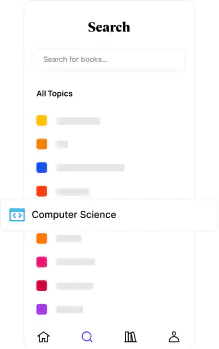📖[PDF] Elements of Partial Differential Equations by Ian N. Sneddon | Perlego
Start your free trial today and explore our endless library.📖 Book - PDF

# Elements of Partial Differential Equations

Ian N. SneddonShare book352 pagesEnglishePUB (mobile friendly) and PDFAvailable on iOS & Android
📖 Book - PDF

# Elements of Partial Differential Equations

Ian N. Sneddon
Book details

Geared toward students of applied rather than pure mathematics, this volume introduces elements of partial differential equations. Its focus is primarily upon finding solutions to particular equations rather than general theory.
Topics include ordinary differential equations in more than two variables, partial differential equations of the first and second orders, Laplace's equation, the wave equation, and the diffusion equation. A helpful Appendix offers information on systems of surfaces, and solutions to the odd-numbered problems appear at the end of the book. Readers pursuing independent study will particularly appreciate the worked examples that appear throughout the text.

## Information

Publisher
Dover Publications
Year
2013
ISBN
9780486162997
Topic
Mathematics
Subtopic
Differential Equations